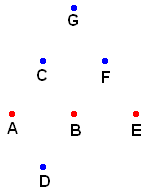## Wednesday, August 27, 2008

### Painted Plane II solution

See the original puzzle

For the first problem, I will first assume that no monochromatic equilateral triangle exists. We start with two points A and B which are the same color (call that color red).By forming equilateral triangles, we can deduce the colors of the following points:
1. Triangle ABC: C is blue
2. Triangle ABD: D is blue
3. Triangle CDE: E is red
4. Triangle BEF: F is blue
5. Triangle AEG: G is blue

Triangle CFG is all blue, contradicting our original assumption. Therefore, there must exist a monochromatic equilateral triangle. QED

For the second problem, I will first assume that there are no singly- or triply-colored equilateral triangles. We start with two points A and B which are the same color (call that color red). Because ABC is an equilateral triangle, C must be a new color, which we will call blue.

At this point, we have to split into several cases:

Case 1: D is green. E is either blue or green, but we can assume without loss of generality that E is green.

Case 2: D is blue. E is green.

Case 3: D is blue. E is red.

For the proof of each case, see this diagram. In each case, the steps go in alphabetical order. In each case you will find a red monochromatic triangle. This contradicts our original assumption. Therefore, there must exist a singly- or triply-colored equilateral triangle. QED

It may interest the reader to know that we can prove a much stronger case of this problem. That is, no matter how many colors we use (as long as there are a finite number of colors), there must always exist a monochromatic equilateral triangle. This is a much harder problem, and it requires a different approach. If you are interested in seeing the proof, stick around and I will eventually post it.